# Statistical Measures¶

The Simulator class collects two list instances with raw data. One list has the length of a session, the other list has the maximum stake during a session. We need to create some descriptive statistics to summarize these stakes and session lengths.

This section presents two ordinary statistical algorithms: mean and standard deviation. Python 3 introduces the statistics module, which simplifies some of the programming required for this chapter. We’ll present the details of how to compute these two statistics for those who are interested in programming fundamentals.

In Statistical Analysis we’ll address the overall goal of gathering and analyzing statistics.

In Some Foundations we’ll look at the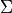operator which is widely used for statistical calculation. We’ll see how to implement this in Python.

In Statistical Algorithms we’ll look specifically at mean and standard deviation.

Since we’re apply statistical calculation to a list of integer values, we’ll look at how we can extend the list in IntegerStatistics Design.

We’ll enumerate the deliverables for this chapter in Statistics Deliverables.

## Statistical Analysis¶

We will design a class with responsibility to retain and summarize a list of numbers and produce the average (also known as the mean) and standard deviation. The Simulator class can then use this this IntegerStatistics class to get an average of the maximum stakes from the list of session-level measures. The Simulator can also apply this IntegerStatistics class to the list of session durations to see an average length of game play before going broke.

We have three design approaches for encapsulating processing:

• We can extend an existing class, or

• We can wrap an existing class, creating a whole new kind of thing, or

• We can delegate the statistical work to a separate function. This is how thing currently stand in Python. We have a build-in list class and a separate statistics module.

A good approach is to extend the built-in list class with statistical summary features. Given this new class, we can replace the original list objects of sample values with a IntegerStatistics instance that both saves the values and computes descriptive statistics.

This design allows us the freedom to switch list implementation classes without making any other changes.

The detailed algorithms for mean and standard deviation are provided in Statistical Algorithms.

## Some Foundations¶

For those programmers new to statistics, this section covers the Sigma operator,.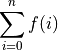Theoperator has three parts to it. Below it is a bound variable, i, and the starting value for the range, written as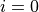. Above it is the ending value for the range, usually something like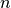. To the right is some function to execute for each value of the bound variable. In this case, a generic function,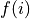is shown. This is read as “sumforin the range 0 to”.

One common definition ofuses a closed range, including the end values of 0 and. However, since this is not a helpful definition for Python, we will defineto use a half-open interval. It has exactlyelements, including 0 and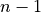; mathematically,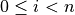. This is how the range() function works, so there are a lot of advantages to using a half-open interval

We prefer the following notation. Since statistical and mathematical texts often used 1-based indexing, some care is required when translating formulae to programming languages that use 0-based indexing.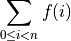Our two statistical algorithms have a form more like the following function. In this we are applying some function,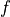, to each value,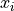, of a list,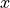.

When computing the mean, as a special case, there is no function applied to the values in the list. When computing standard deviation, the function involves subtracting and multiplying.

## Python Coding¶

Consider we look at the following summation: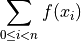We can transform this definition directly into a for statement that sets the bound variable to all of the values in the range, and does some processing on each value of the list of integers,.

This is the Python implementation of Sigma. This computes two values, the sum, s and the number of elements, n.

s = sum(theList)
n = len(theList)


When computing the standard deviation, we do something that has the following overall pattern:

s = sum(f(x) for x in theList)
n = len(theList)


Where the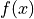calculation computes the measure of deviation from the average.

## Statistical Algorithms¶

We’ll look at two important algorithms:

### Mean¶

Computing the mean of a list of values, x, is relatively simple. The mean is the sum of the values divided by the number of values in the list. Here’s the formal definition of the mean,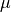, of a list of values,. There areitems in the list,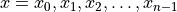.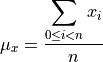The definition of themathematical operator leads us to the following method for computing the mean:

sum(self)/len(self)


This matches the mathematical definition nicely.

### Standard Deviation¶

The standard deviation can be done a few ways. We’ll use the formula shown below. This computes a deviation measurement as the square of the difference between each sample and the mean.

The sum of these measurements is then divided by the number of values times the number of degrees of freedom to get a standardized deviation measurement.

Again, the formula summarizes the for statement, so we’ll show the formula followed by an overview of the code.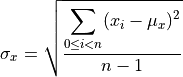The definition of themathematical operator leads us to the following method for computing the standard deviation:

We can use a generator expression to compute the squares of the deviations from the mean. The expression (x-m)**2 for x in self is a sequence of values. The sum() function can sum this sequence.

m = mean(x)
math.sqrt(sum((x-m)**2 for x in self) / (len(self)-1))


This seems to match the mathematical definition nicely.

## IntegerStatistics Design¶

class IntegerStatistics(list)

IntegerStatistics computes several simple descriptive statistics of int values in a list.

This extends list with some additional methods.

### Constructors¶

Since this class extends the built-in list, we’ll leverage the existing constructor.

### Methods¶

IntegerStatistics.mean(self) → float

Computes the mean of the List of values.

IntegerStatistics.stdev(self) → float

Computes the standard deviation of the List values.

## Statistics Deliverables¶

There are three deliverables for this exercise. These classes will include the complete Python docstring.

Here is some standard deviation unit test data.

 Sample Value 10 8 13 9 11 14 6 4 12 7 5

Here are some intermediate results and the correct answers given to 6 significant digits. Your answers should be the same to the precision shown.

sum

99

count

11

mean

9.0

sum (x-m)**2

110.0

stdev

3.317

## Looking Forward¶

The key feature of this simulator is introducing different kinds of betting strategies. Let’s look at a player that bets at random. It’s important to compare random betting against the persistent better of the Passenger57 class. The house edge is the same and the performance of the two strategies should be very similar.